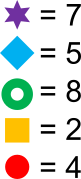#### You may also like### Special Numbers

My two digit number is special because adding the sum of its digits to the product of its digits gives me my original number. What could my number be?Think of a number and follow the machine's instructions... I know what your number is! Can you explain how I know?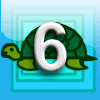### First Forward Into Logo 6: Variables and Procedures

Learn to write procedures and build them into Logo programs. Learn to use variables.

# Shape Products

##### Age 11 to 14 Challenge Level:

Thank you to everyone who sent in a solution. There were too many good solutions to mention everybody here, but this is a selection of what we received.

Jessica from Royds School in the UK used a trial approach to solve the problem:

We started trying numbers for the blue rectangle. We found that 3 worked and then we used this to fill the rest of the numbers.  We wrote a list a list of the numbers at the top of the page to see which we had used.

Siri and Amelie from Priestlands School in the UK used a systematic method to make sense of the equations. They said:
We used a trial and error technique to find out which shapes were what - by writing out the numbers 0 to 12 by each shape, we were able to cross out the numbers which we knew were impossible for our shape to be, eventually leaving us with the answer.

Anny from St Mary's School, Cambridge in the UK sent in this solution, using factors to find the values of the shapes.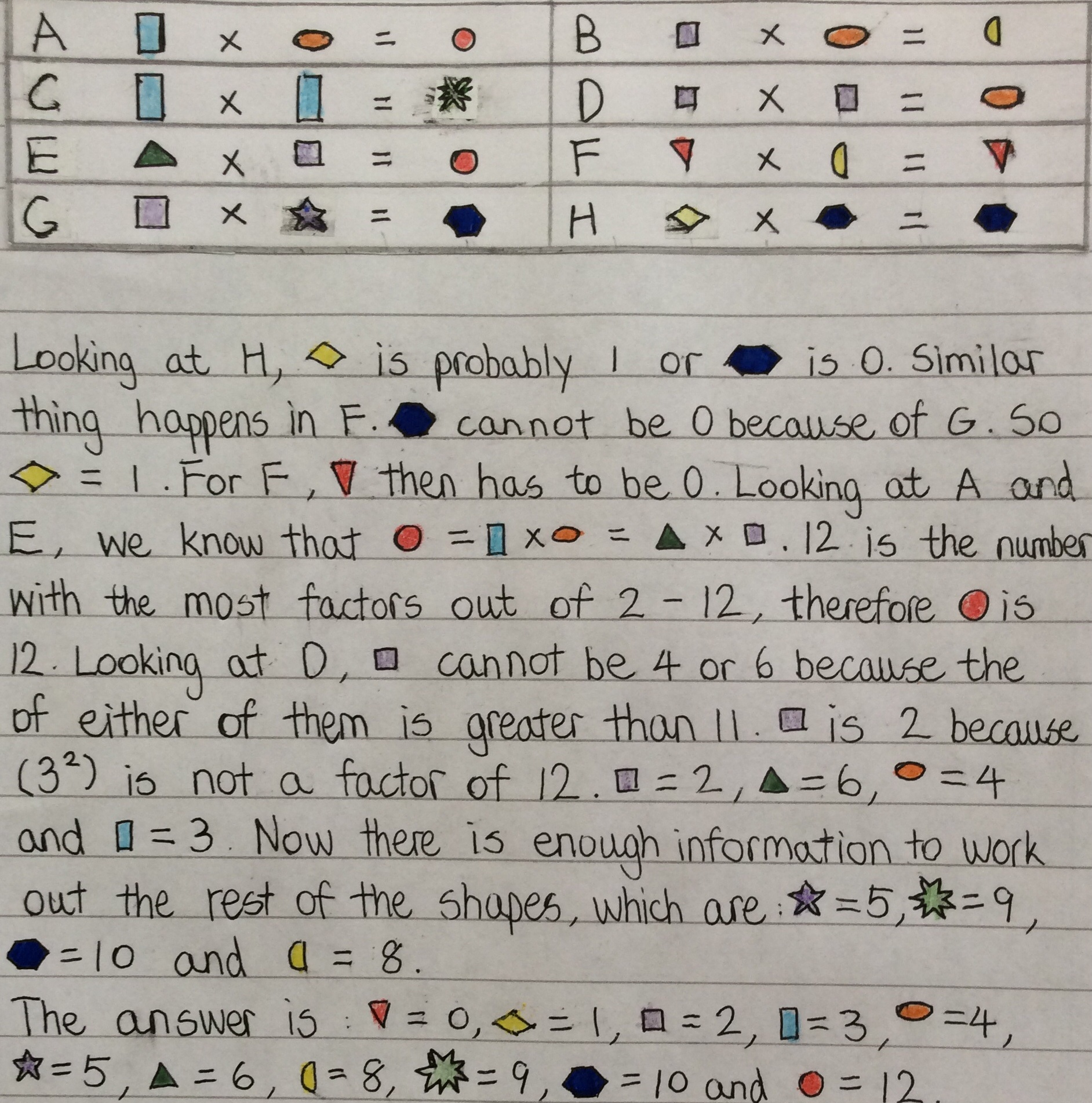Abi from The Grange School, William from Methodist College and Tia from North London Collegiate School, all in the UK, began by looking at the equations which involved squaring. Abi said:
I first noticed that the products of equations C and D are both square numbers. Since the products of both are different to the factors, this rules out 1 and so there are only two possible square numbers between 0 and 12: 4 and 9. Therefore the oval and the star each equal 4 or 9, and the rectangle and the square each equal 2 or 3.

Equation B involves the two different integers from equation D. The two possible answers must be [2 $\times$ 4 = 8] or [3 $\times$ 9 = 27]. 27 isn't a number between 0 and 12, so this can't be the correct equation. Instead, we know that the purple square is equal to 2, the orange oval is equal to 4, and the yellow semicircle is equal to 8. This also means that equation C is [3 $\times$ 3 = 9]. We can substitute these numbers into other equations A,E,F,G.

Equations F and H have the same product as one of its factors. This means that one of the factors in each equation has to be either 1 or 0. In equation F, we know that [a $\times$ 8 = a]. The red triangles (a) have to be 0 because anything multiplied by 0 is 0. I also know that it can't be 1 as [1 $\times$ 8 = 8]. These numbers are the wrong way round for this equation to work. 1 must therefore fit in equation H as the yellow diamond.

As I have substituted some shapes in the equations for numbers I already know, equation A is left as [3 $\times$ 4 = a]. a (or the red circle) is easy to find to be 12. 12 is also the solution to equation E: [a $\times$ 2 = 12]. By making a the subject, we know that [a = 12/2 = 6].

We are now left with unknown numbers in only equations G and H. G says [2 $\times$ a = c]. This means that c is an even number. The numbers remaining to choose from are 5,7,10 and 11, of which 10 is the only even number. This is the blue hexagon. By changing equation G to make the purple star the subject, we know that [a = 10/2 = 5].

Vid from Primary school Loka Črnomelj in Slovenia sent in a very clear solution which uses a mixture of the two methods above. You can see Vid's solution herevid.pdf

Sophie, Amelia and Natasha from Holy Trinity CE Academy, Rothwell in the UK sent in their work on the extension. This is how they solved the problem: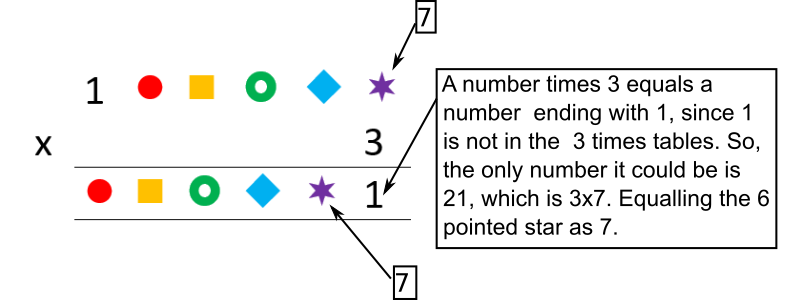After figuring out the first number, we decided to work our way along the line of shapes.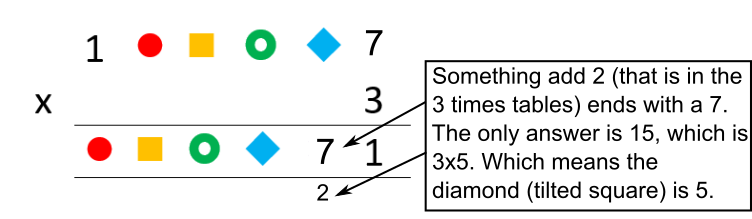After figuring out how to work out each shape's number, these were our answers: